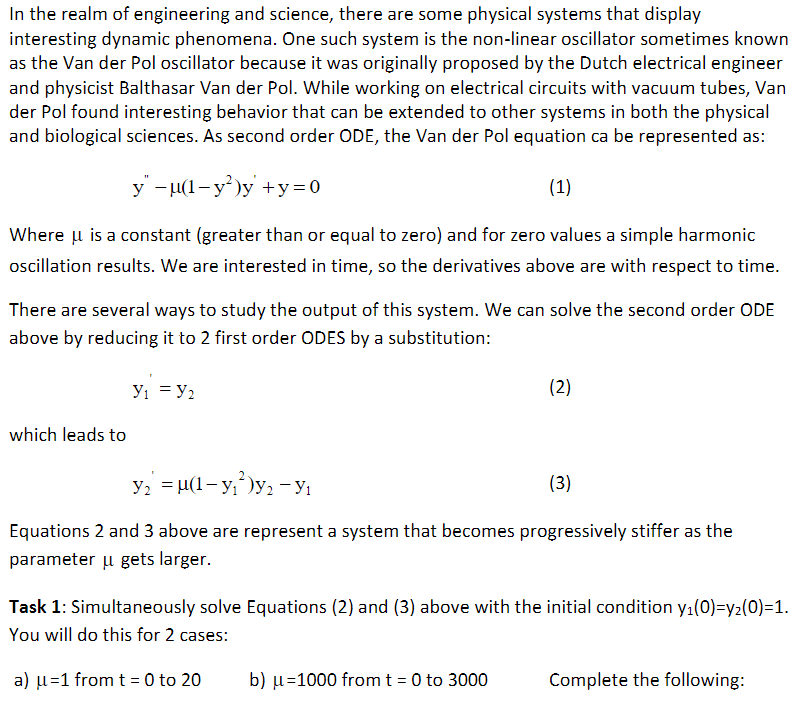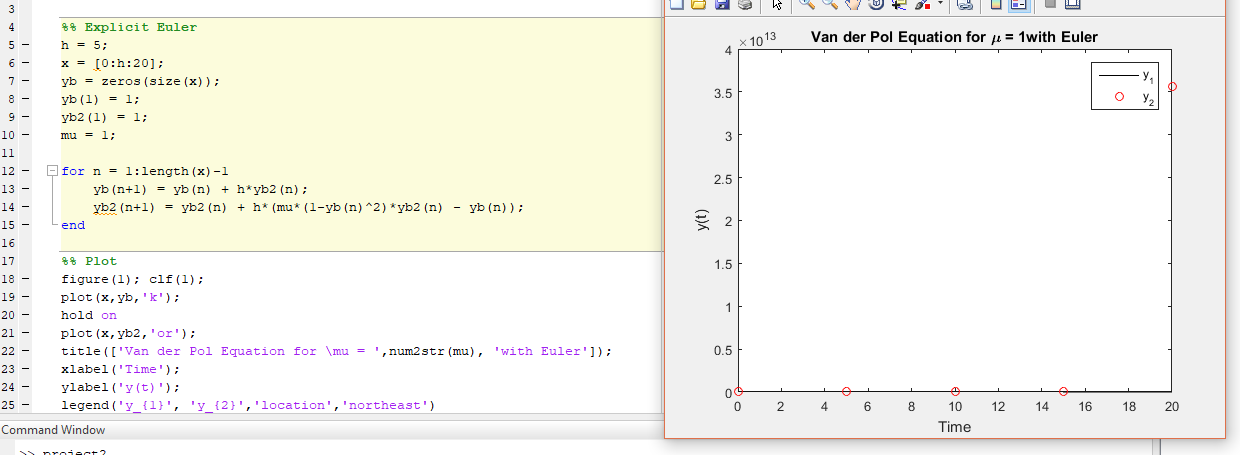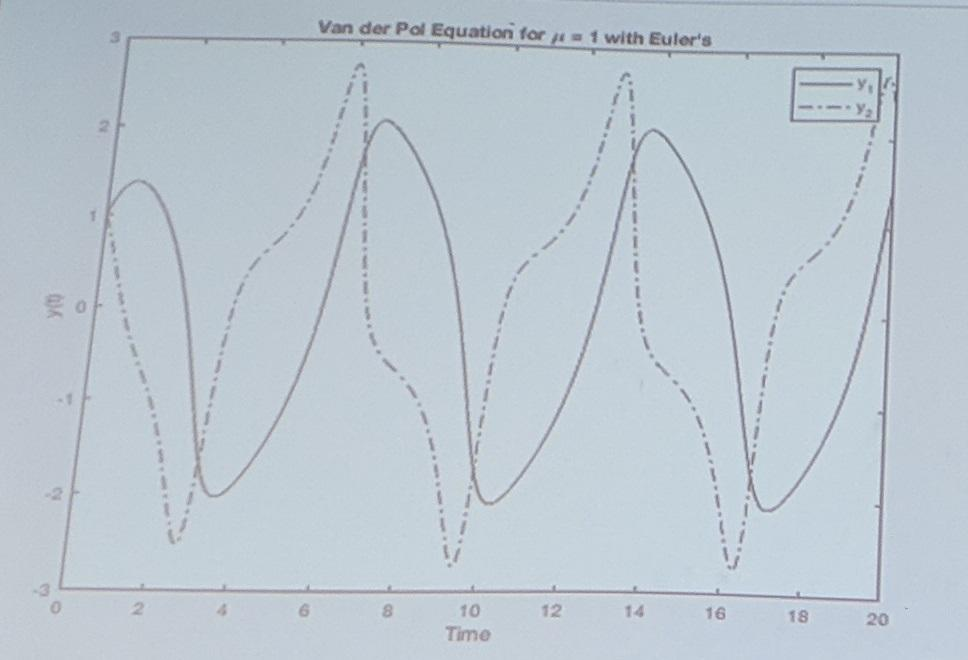# Euler's Method for stiff ODE

15 ビュー (過去 30 日間)
jake thompson 2018 年 3 月 1 日
Commented: Rena Berman 2019 年 12 月 12 日
Having problem with my code, I'm trying to make my graph (the middle) look like the bottom graph. I feel like it is something with the initial inputs, I pretty sure my for loop is correct. Thanks for the help I appreciate it!if true
% %%Explicit Euler
h = 5;
x = [0:h:20];
yb = zeros(size(x));
yb(1) = 1;
yb2(1) = 1;
mu = 1;
for n = 1:length(x)-1
yb(n+1) = yb(n) + h*yb2(n);
yb2(n+1) = yb2(n) + h*(mu*(1-yb(n)^2)*yb2(n) - yb(n));
end
%%Plot
figure(1); clf(1);
plot(x,yb,'k');
hold on
plot(x,yb2,'or');
title(['Van der Pol Equation for \mu = ',num2str(mu), 'with Euler']);
xlabel('Time');
ylabel('y(t)');
legend('y_{1}', 'y_{2}','location','northeast')
end#### 4 件のコメント

jake thompson 2018 年 3 月 1 日
That's what I figured also but everytime I change my step size every thing gets a bit strange.
Torsten 2018 年 3 月 1 日
Could you show the plot with stepsize h=0.01, say ?
Rena Berman 2019 年 12 月 12 日

サインイン to comment.

### 採用された回答

Jim Riggs 2018 年 3 月 1 日

Torsten is right. There is no way you will get a solution with a large step size (h=5). I checked out your code and it works fine for mu=1 with a step size of h=0.1
I would recommend the following two changes;
clear x yb yb2
at the start of the script.
2) I would also recommending pre-allocating the yb2 vector. you can do this by changing
yb2(1) = 1
to
yb2 = yb.
this will make yb2 and yb the same size, and they will both have a 1 in the first position.
Now, as you increase the value of mu, the system becomes more stiff. For example, using a step size of h=0.1 I can get a solution for mu =2 and mu=3, but at mu=4 the function blows up after about 14 seconds. (it is numerically unstable). Now you have to reduce the step size to improve the calculation stability. In order to get a solution with mu=1000, and to execute for 3000 seconds without blowing up, the step size must be extremely small (10^-6 or even smaller). My problem is that this is taxing the capabilities of my computer and I cannot find the solution.
A major part of the problem is the use of the Euler integration method. This method is not well suited for stiff systems (perhaps that is what this lesson is about). For this problem, I would switch to a Runge-Kutta integrator and try my favorite [5,6] Runge Kutta method. This would allow the use of a much larger step size (probably 3 orders of magnitude) compared to what is required for Euler integration.

#### 2 件のコメント

jake thompson 2018 年 3 月 2 日
I edited the first few lines of my code and solved my problem!
if true
m = 500; % number of steps
t_span = [0 20]; %time span
h = t_span(end)/(m-1);
x = [0:h:t_span(end)];
end
Jim Riggs 2018 年 3 月 2 日
This will work for mu=1, since it results in a step size of 0.04. Bit it will not work for significantly larger values of mu. As mu increases, you still need to decrease the step size. You will have to experiment to find acceptable values. And when you increase the timespan, the step size gets bigger. This is also not desirable.

サインイン to comment.